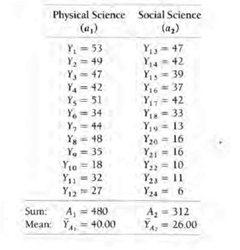# ANOVA Statistical Concept

ANOVA Statistical Concept

“Analysis of variance (ANOVA) was developed by Ronald A. Fisher in the 1930s (although the name “analysis of variance” came later from John W. Tukey)” (Salkind, 2007 para 1). I am going to quote and refer to Keppel and Zedeck (1989) to explain the ANOVA concept “Suppose we examined the data from an experiment in which two groups received different treatments but we did not take this fact into consideration in our analysis. Although we could calculate a mean and a standard deviation to describe the total data set, they would not be particularly useful, since this summary would obscure any effects that the two different treatments might have” (p.71)

We run ANOVA when we want to compare the effects of any treatment or condition between two groups.  For example, we can have fifth grade students who were exposed to a set of 60 new vocabulary words in two different context lectures: one of physical science and one on social science. After the lectures the students were tested on vocabulary, and the study is trying to determine which of the two groups scored a better performance on the vocabulary test.

For the experiment, the researcher creates 24 subjects randomly selected and then he also randomly separates the groups in two groups of 12. One will be lectured on social sciences and the other with physical sciences. At the end of the experiment, the researcher will examine which of the two groups score better on the number of new words. Without getting into the specifics of the ANOVA calculation (A good exercise to research, since there are plenty of explanations on the ANOVA test) notice the table below with the specifics scores and the mean for the two groups. Be aware that it is not enough to say that the mean of group one (40) is better than the mean of group two (26) we must proceed with the calculation and determine if that difference is significant (at whatever significant level you are testing)So precisely, ANOVA is the statistical procedure to compare the two-means using the sum of squares concepts and determine statistically which of the two groups score better. Notice that the NULL hypothesis will say that there is ‘no difference’ in the scores of the two groups.

References

Keppel, G., & Zedeck, S. (1989). Data Analysis for research designs. New York: W. H. Freeman and Company.

Salkind, N. (2007). Encyclopedia of Measurement and Statistics. doi:10.4135/9781412952644Additional content will be provided upon request.